Open in App
Not now

# Decision Tree Introduction with example

• Difficulty Level : Medium
• Last Updated : 02 Feb, 2023

Decision tree algorithm falls under the category of supervised learning. They can be used to solve both regression and classification problems. Decision tree uses the tree representation to solve the problem in which each leaf node corresponds to a class label and attributes are represented on the internal node of the tree. We can represent any boolean function on discrete attributes using the decision tree.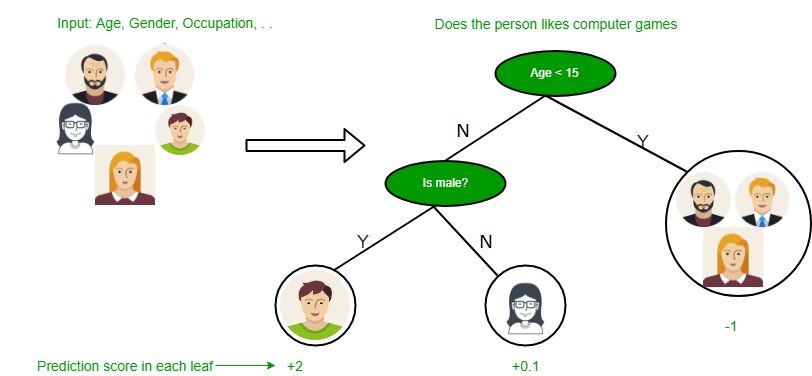Below are some assumptions that we made while using the decision tree:

• At the beginning, we consider the whole training set as the root.
• Feature values are preferred to be categorical. If the values are continuous then they are discretized prior to building the model.
• On the basis of attribute values, records are distributed recursively.
• We use statistical methods for ordering attributes as root or the internal node.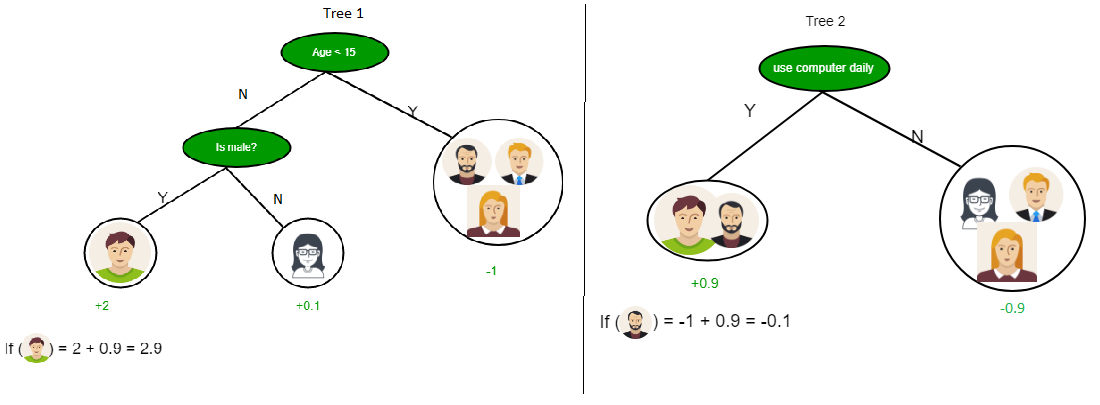As you can see from the above image the Decision Tree works on the Sum of Product form which is also known as Disjunctive Normal Form. In the above image, we are predicting the use of computer in the daily life of people. In the Decision Tree, the major challenge is the identification of the attribute for the root node at each level. This process is known as attribute selection. We have two popular attribute selection measures:

1. Information Gain
2. Gini Index

1. Information Gain When we use a node in a decision tree to partition the training instances into smaller subsets the entropy changes. Information gain is a measure of this change in entropy. Definition: Suppose S is a set of instances, A is an attribute, Sv is the subset of S with A = v, and Values (A) is the set of all possible values of A, thenEntropy Entropy is the measure of uncertainty of a random variable, it characterizes the impurity of an arbitrary collection of examples. The higher the entropy more the information content. Definition: Suppose S is a set of instances, A is an attribute, Sv is the subset of S with A = v, and Values (A) is the set of all possible values of A, thenExample:

For the set X = {a,a,a,b,b,b,b,b}
Total instances: 8
Instances of b: 5
Instances of a: 3
Entropy H(X)  = -\left [ \left ( \frac{3}{8} \right )log_{2}\frac{3}{8} + \left ( \frac{5}{8} \right )log_{2}\frac{5}{8} \right ]

= -[0.375 * (-1.415) + 0.625 * (-0.678)]
= -(-0.53-0.424)
=  0.954

Building Decision Tree using Information Gain The essentials:

• Use info gain to choose which attribute to label each node with
• Note: No root-to-leaf path should contain the same discrete attribute twice
• Recursively construct each subtree on the subset of training instances that would be classified down that path in the tree.
• If all positive or all negative training instances remain, the label that node “yes” or “no” accordingly
• If no attributes remain, label with a majority vote of training instances left at that node
• If no instances remain, label with a majority vote of the parent’s training instances.

Example: Now, let us draw a Decision Tree for the following data using Information gain. Training set: 3 features and 2 classes

Here, we have 3 features and 2 output classes. To build a decision tree using Information gain. We will take each of the features and calculate the information for each feature.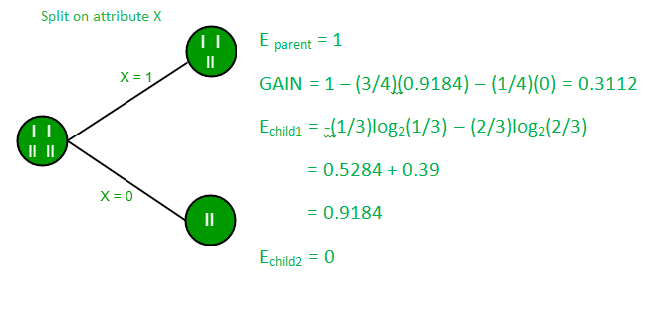Split on feature X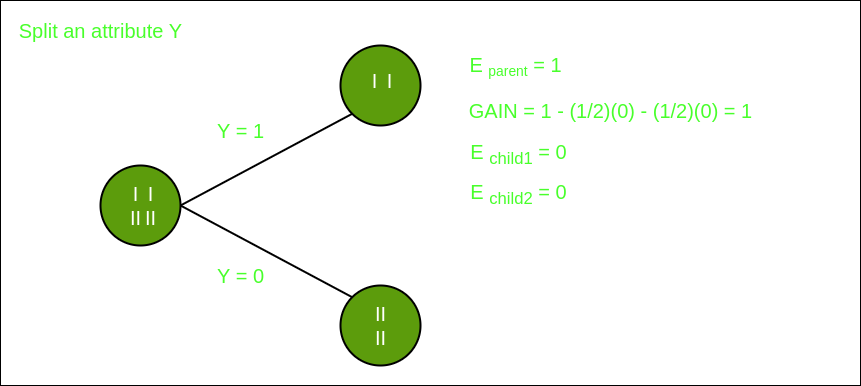Split on feature Y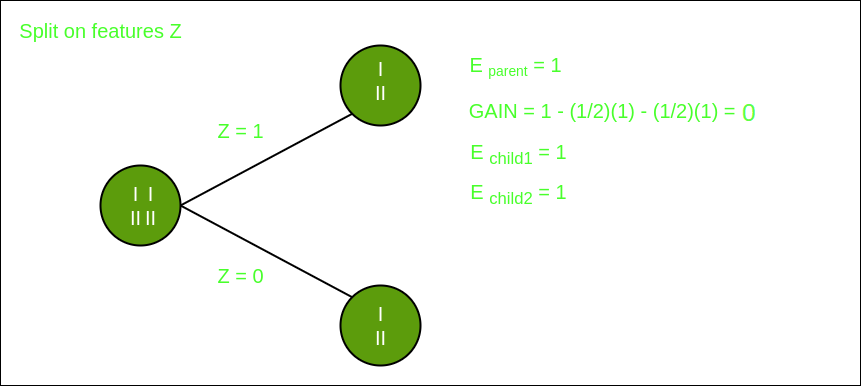Split on feature Z

From the above images, we can see that the information gain is maximum when we make a split on feature Y. So, for the root node best-suited feature is feature Y. Now we can see that while splitting the dataset by feature Y, the child contains a pure subset of the target variable. So we don’t need to further split the dataset. The final tree for the above dataset would look like this: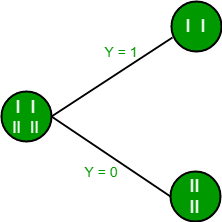2. Gini Index

• Gini Index is a metric to measure how often a randomly chosen element would be incorrectly identified.
• It means an attribute with a lower Gini index should be preferred.
• Sklearn supports “Gini” criteria for Gini Index and by default, it takes “gini” value.
• The Formula for the calculation of the Gini Index is given below.
My Personal Notes arrow_drop_up
Related Articles